# PRGC: Potential Relation and Global Correspondence Based Joint Relational Triple Extraction

## PRGC

PRGC(Potential Relation and Global Correspondence)的模型特别简单, 简单到一张图就能说的清楚: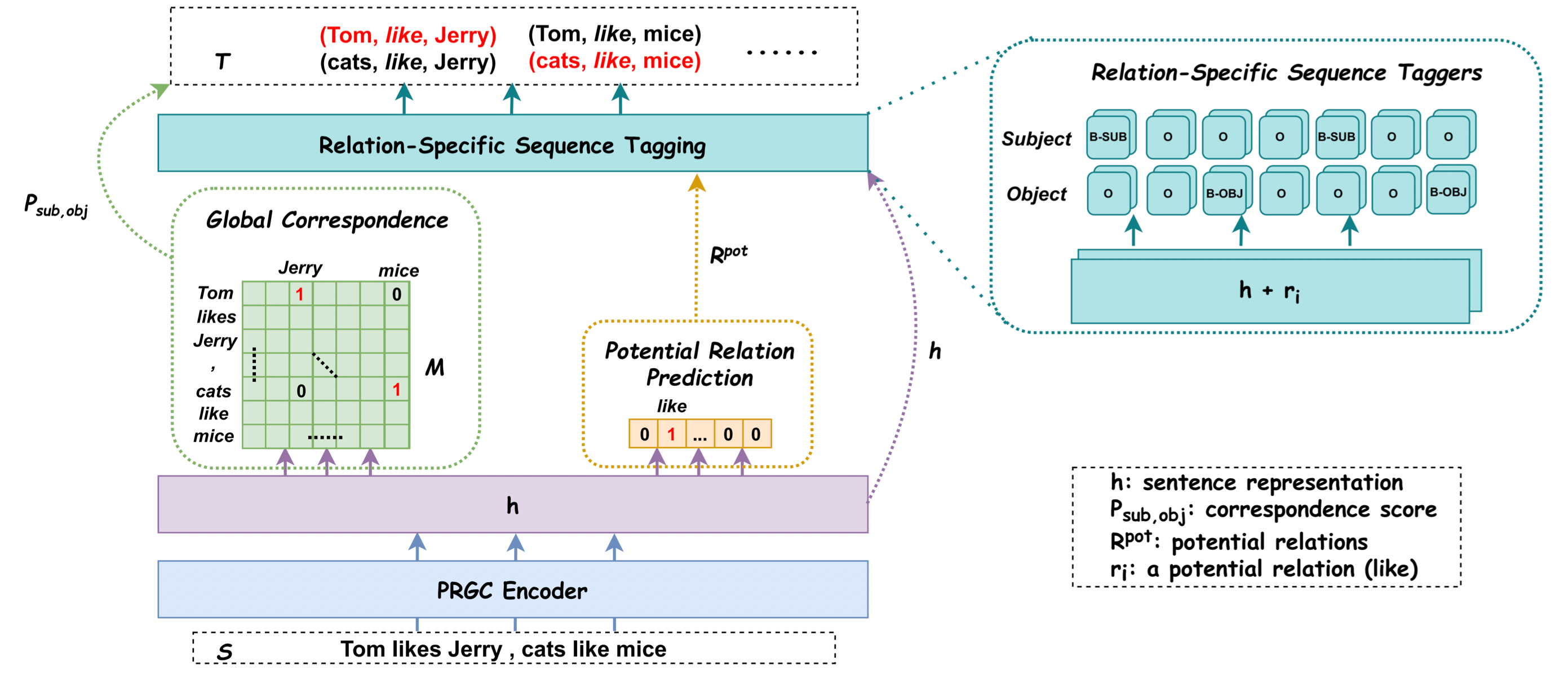### Problem Definition

• Relation Judgement: 检测句子$S$ 中的所有潜在关系$Y_r(S) = \set{r_1, r_2, \dots, r_m \mid r_i \in R}$, $m$ 为潜在关系数量.
• Entity Extraction: 抽取出句子$S$ 在潜在关系$r_i$ 下的实体, 用BIO标注, 即$Y_e(S, r_i \mid r_i \in R) = \set{t_1, t_2, \dots, t_n}$, 其中$t_j$ 为Tag.
• Subject - Object Alignment: 找到句子$S$ 中的头尾实体关系类型无关全局对应关系, 用矩阵$\mathbf{M}$ 来表示, $Y_s(S) = \mathbf{M} \in \mathbb{R}^{n\times n}$.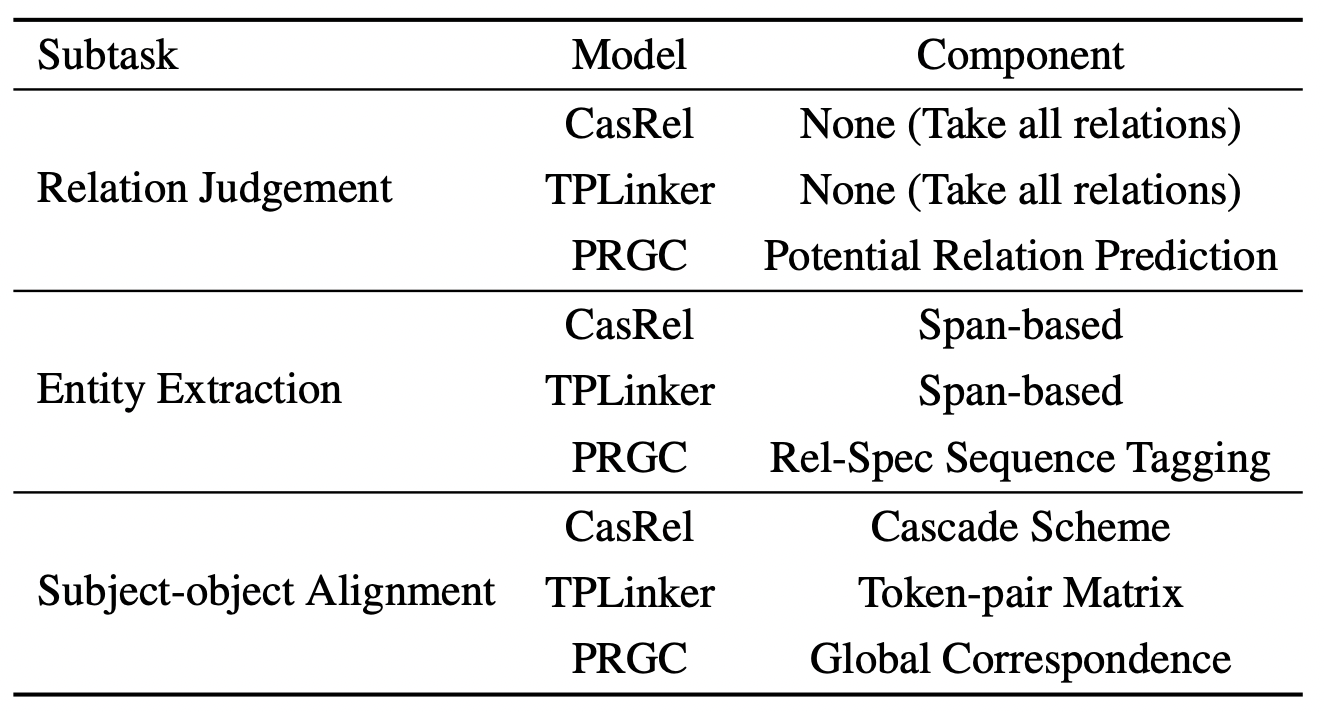### PRGC Encoder

$$Y_{enc}(S)= \set{h_1, h_2, \dots, h_n \mid h_i \in \mathbb{R}^{d\times1}}$$

### PRGC Decoder

Decoder才是模型的主要部分.

#### Potential Relation Prediction

\begin{aligned} \mathbf{h}^{a v g} &=\operatorname{Avgpool}(\mathbf{h}) \in \mathbb{R}^{d \times 1} \\ P_{r e l} &=\sigma\left(\mathbf{W}_{r} \mathbf{h}^{a v g}+\mathbf{b}_{r}\right) \end{aligned}

#### Relation - Specific Sequence Tagging

$$\begin{array}{r} \mathbf{P}_{i, j}^{s u b}=\operatorname{Softmax}\left(\mathbf{W}_{s u b}\left(\mathbf{h}_{i}+\mathbf{u}_{j}\right)+\mathbf{b}_{s u b}\right) \\ \mathbf{P}_{i, j}^{o b j}=\operatorname{Softmax}\left(\mathbf{W}_{o b j}\left(\mathbf{h}_{i}+\mathbf{u}_{j}\right)+\mathbf{b}_{o b j}\right) \end{array}$$

#### Global Correspondence

$$P_{i_{s u b}, j_{o b j}}=\sigma\left(\mathbf{W}_{g}\left[\mathbf{h}_{i}^{s u b} ; \mathbf{h}_{j}^{o b j}\right]+\mathbf{b}_{g}\right)$$

### Training Strategy

\begin{aligned} \mathcal{L}_{r e l}&=-\frac{1}{n_{r}} \sum_{i=1}^{n_{r}}\left(y_{i} \log P_{r e l}+\left(1-y_{i}\right) \log \left(1-P_{r e l}\right)\right) \\ \mathcal{L}_{s e q}&=-\frac{1}{2 \times n \times n_{r}^{p o t}} \sum_{t \in\{s u b, o b j\}} \sum_{j=1}^{n_{r}^{p o t}} \sum_{i=1}^{n} \mathbf{y}_{i, j}^{t} \log \mathbf{P}_{i, j}^{t} \\ \mathcal{L}_{g l o b a l}&=-\frac{1}{n^{2}} \sum_{i=1}^{n} \sum_{j=1}^{n}\left(y_{i, j} \log P_{i_{s u b}, j_{o b j}} +\left(1-y_{i, j}\right) \log \left(1-P_{i_{s u b}, j_{o b j}}\right)\right) \end{aligned}

Potential Relation Prediction和Global Correspondence都可以看做是多标签二分类问题, 所以都用二分类交叉熵优化. 而Relation - Specific Sequence Tagging和NER大多数一致, 视为序列标注问题, 使用多分类交叉熵优化.

$$\mathcal{L}_{\text {total }}=\alpha \mathcal{L}_{r e l}+\beta \mathcal{L}_{s e q}+\gamma \mathcal{L}_{\text {global }}$$

$\alpha, \beta, \gamma$ 为超参, 作者在这里简单的设它们三个均为1.

## Experiments and Analysis

### Datasets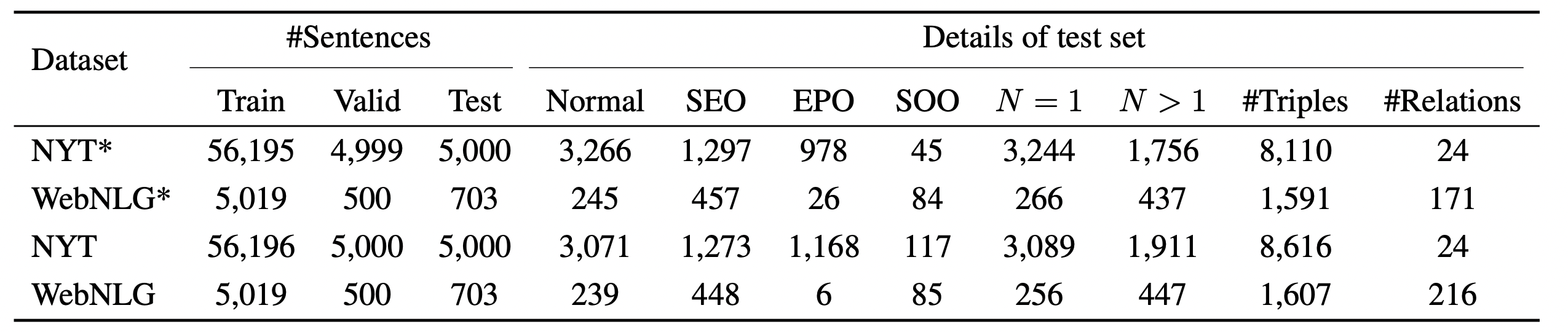### Experimental Results

#### Main Results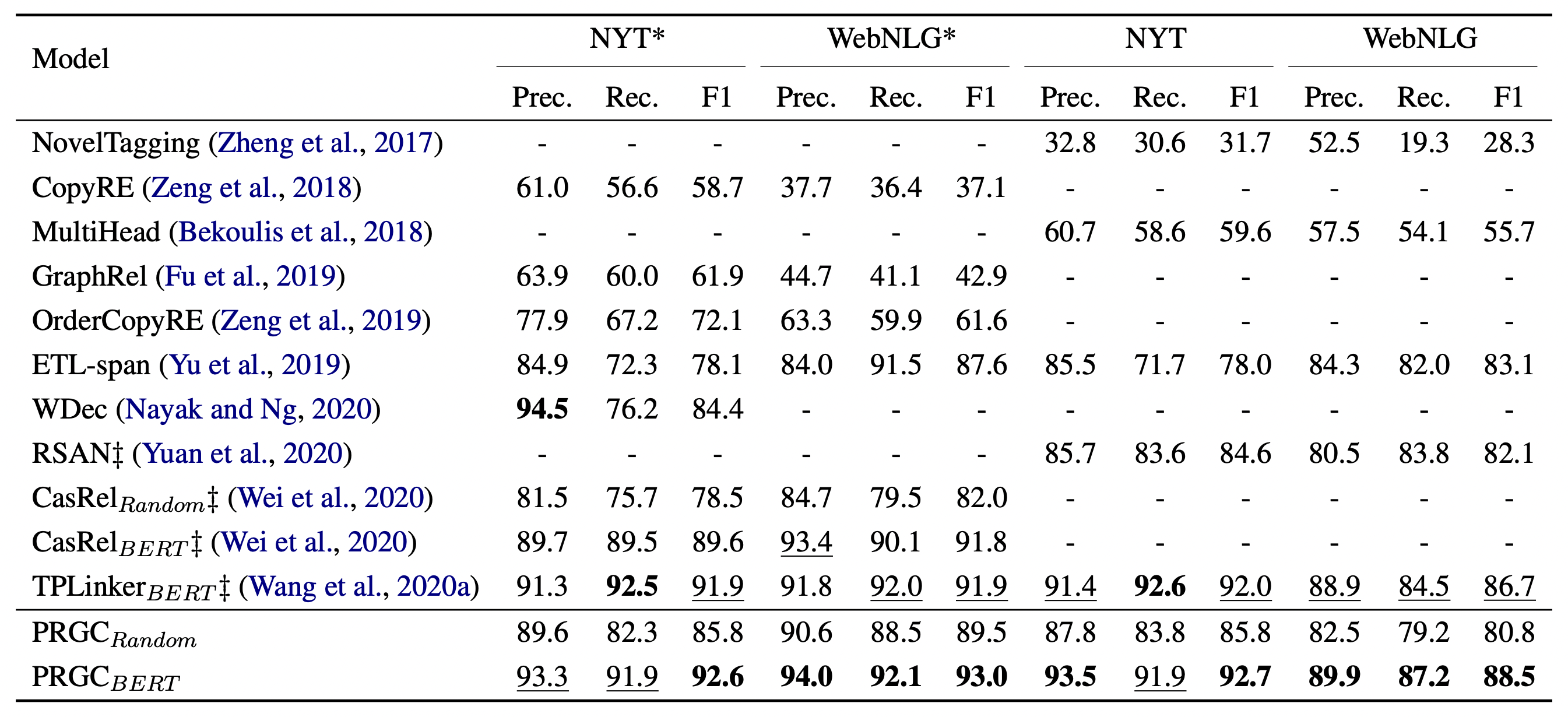#### Detailed Results on Complex Scenarios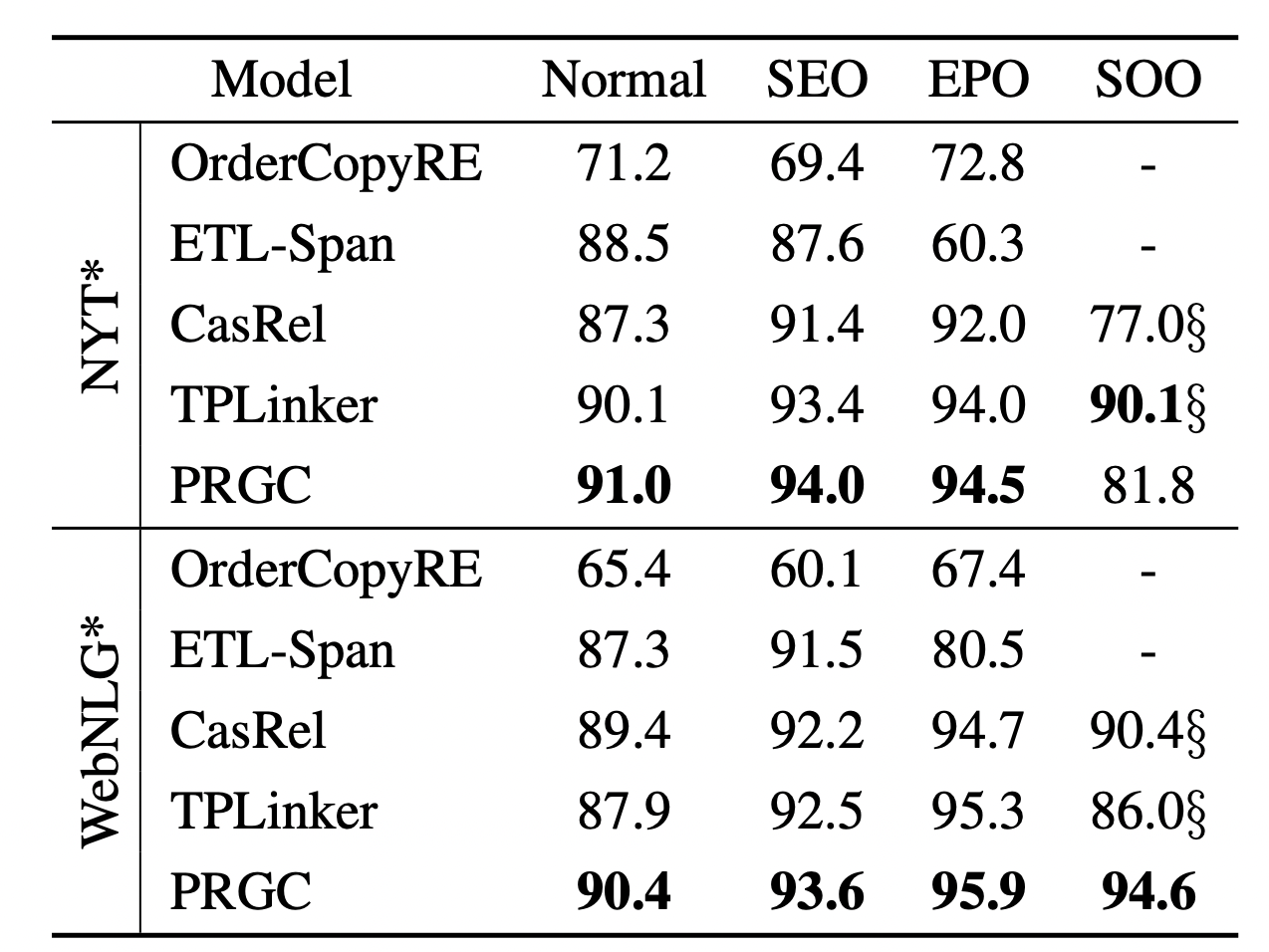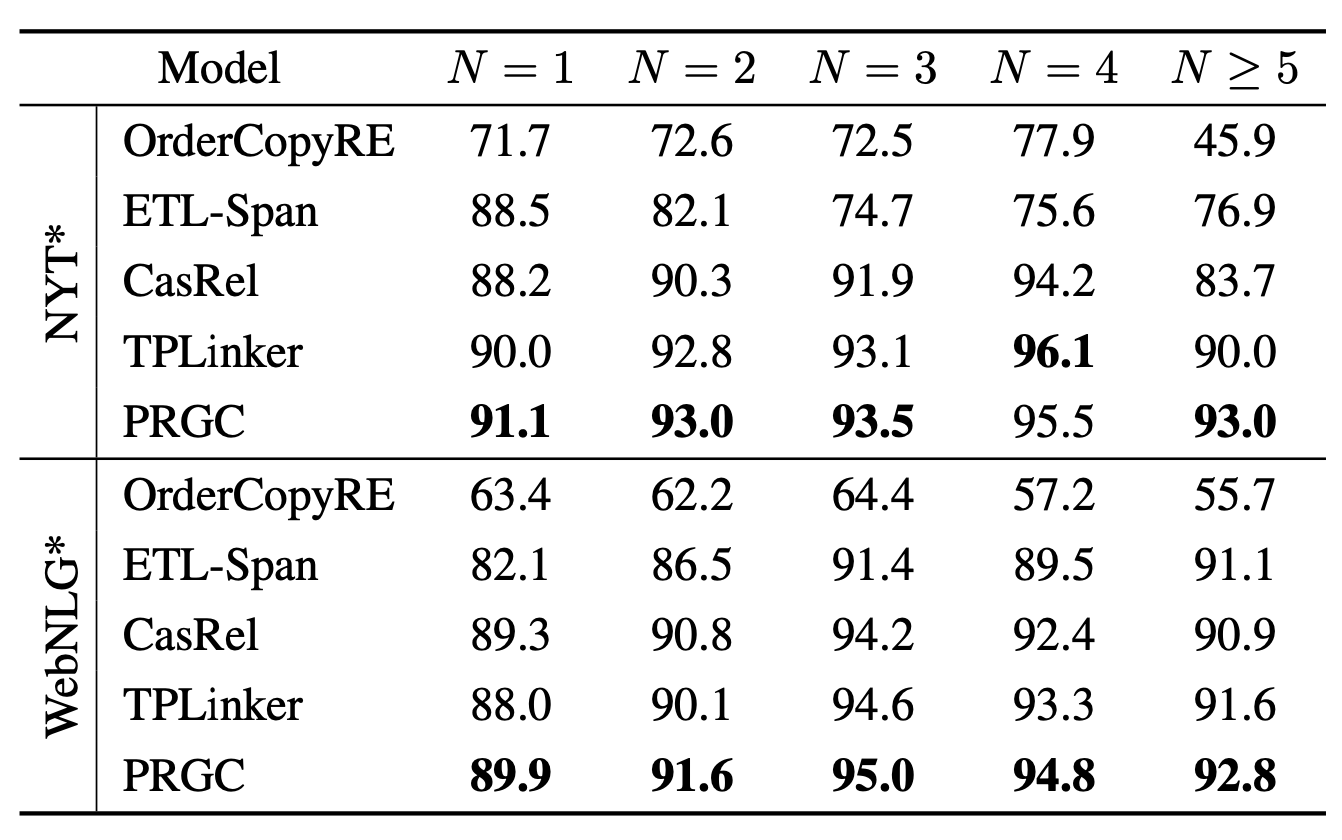PRGC各数量三元组均有不错的抽取性能.

### Analysis

#### Model Efficiency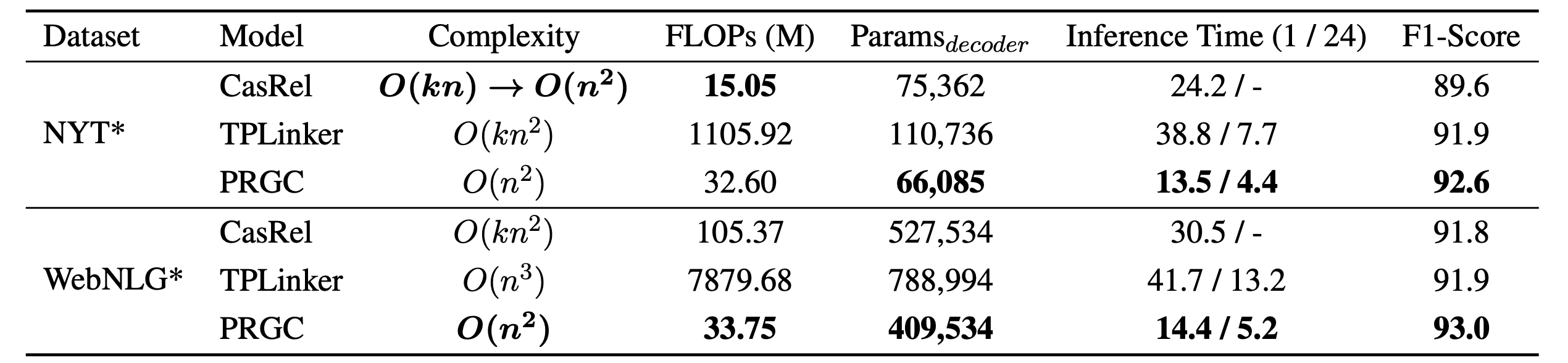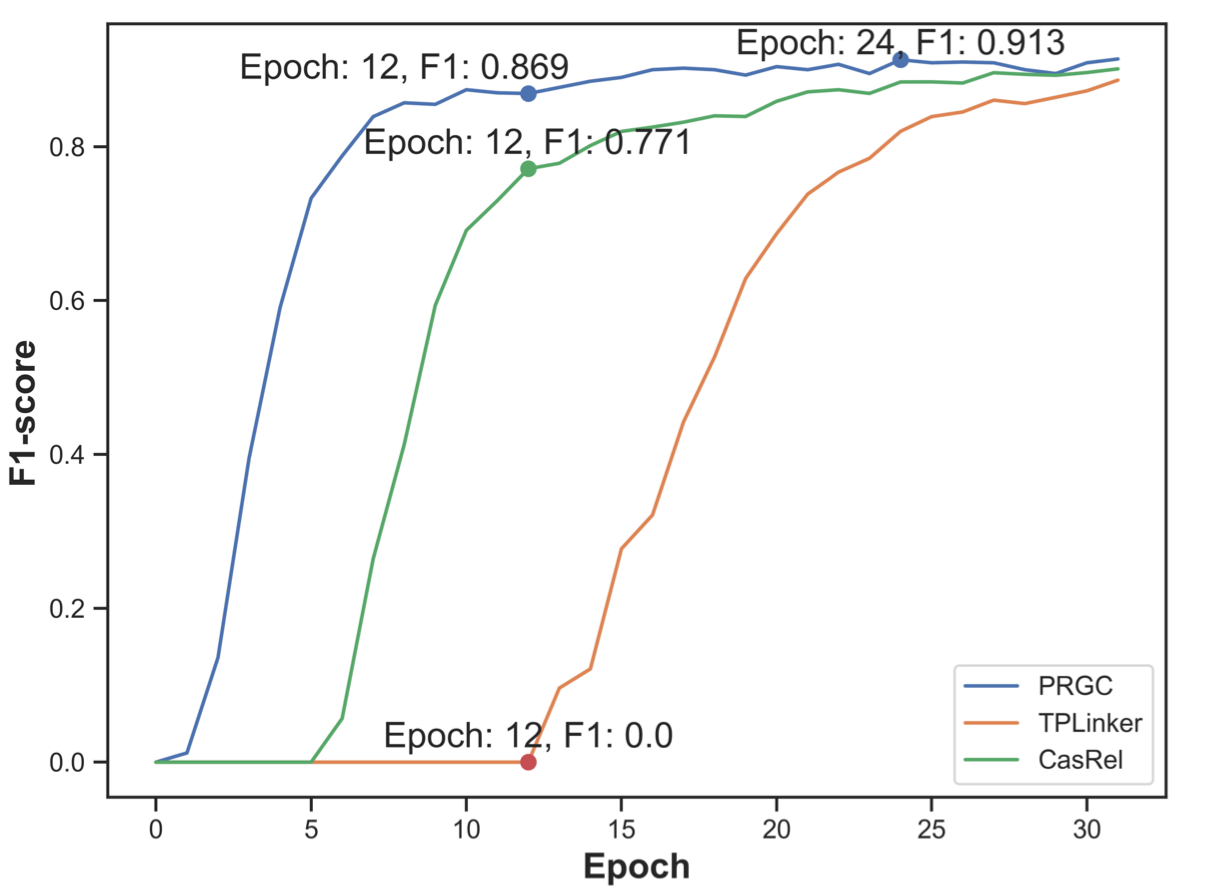#### Ablation Study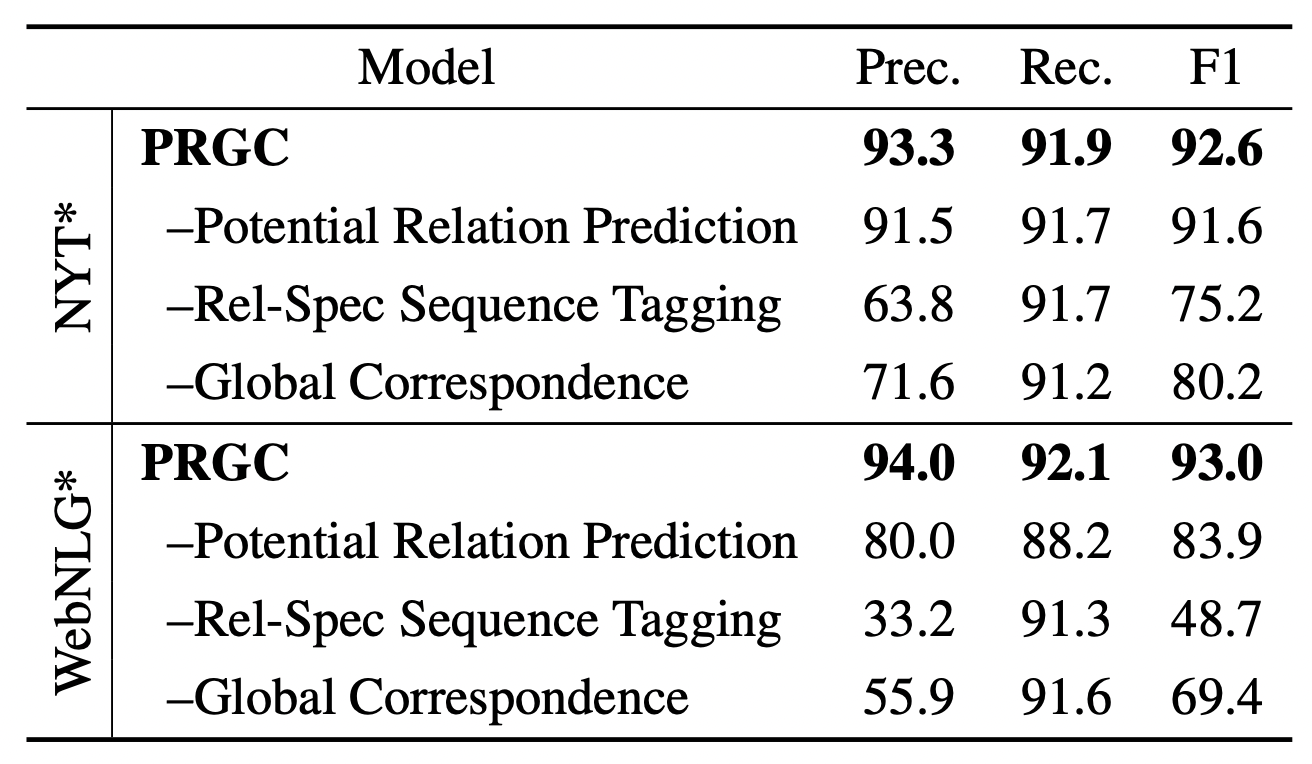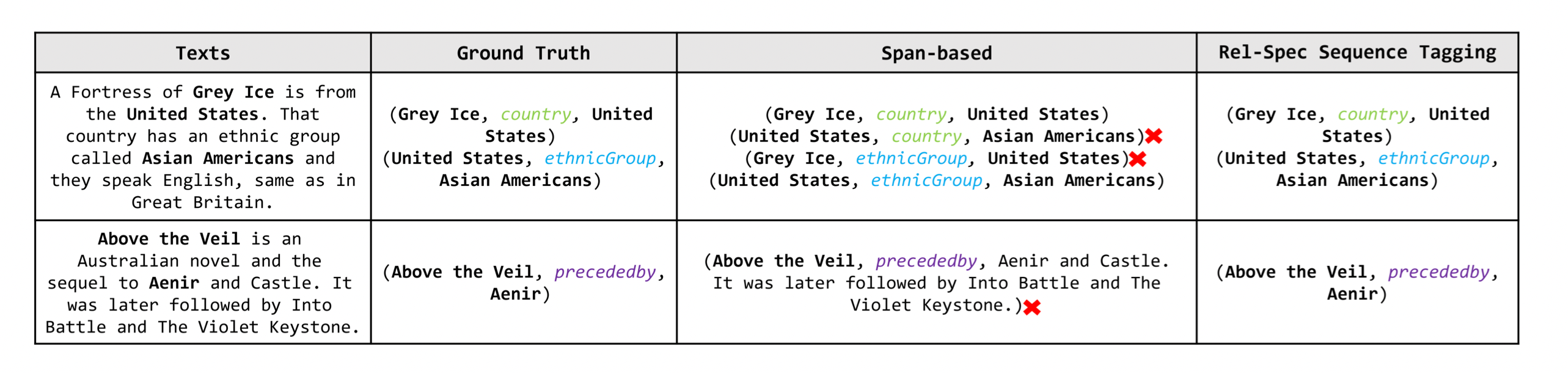• Potential Relation Prediction: 去掉该组件后, 不再过滤冗余关系, 而是采用全关系预测. 关系数量比较少的NYT受影响不大, 而WebNLG下降的比较多. 由此说明该组件有良好的过滤冗余关系作用.

其实相对后面两个组件来说这个组件影响并不够大, 可能比较强的点在加速上.

• Rel - Spec Sequence Tagging: 将BIO的标注方式替换为抽取实体Span的标注方式后, 精度骤减. 联合Case可以说明该模块使得模型更倾向于关注语义而非关注实体位置, 并且更关注关系.

• Global Correspondence: 去掉该组件, 改用启发式最近匹配原则构成三元组, 对精度有很大影响.

Global Correspondence能保证前面两个组件精度低的情况下, 最后组成三元组时的精度, 就怕前面漏标(召回低).

## Summary

PRGC把任务拆解为三个子任务: 潜在关系预测, 关系特化的实体标注, 头尾实体对齐, 并按照三个子任务设计了相应的三个模块, 简单明了, 直接有效. 整体来说模型并不复杂, 论文包装的比较好, 给每个模块都找到了相应的解释.

上一篇GRTE: A Novel Global Feature-Oriented Relational Triple Extraction Model based on Table Filling

2022-06-02T5: Exploring the Limits of Transfer Learning with a Unified Text-to-Text Transformer

2022-04-22
目录